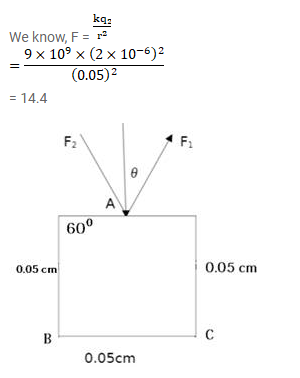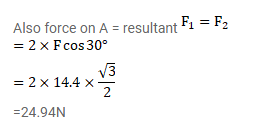# Three equal charges , 2.0 ×10-6 C each,`
Question:

Three equal charges, $2.0 \times 10^{-6} \mathrm{C}$ each, are held fixed at the three corners of an equilateral triangles of side $5 \mathrm{~cm}$. Find the coulomb force experienced by one of the charges due to the rest two.

Solution: